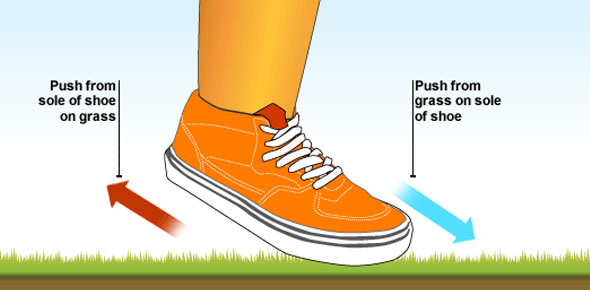Chapter 10 Quiz - Motion (Holt)

10 Questions | Total Attempts: 263SettingsMotion is the action or process of a physical body being moved. There is a way in which one can calculate speed and we covered it in class as we tried to understand the laws of motion and how to calculate how far or fast an object may move. Do you believe you fully understood chapter 10 section one on measuring motion? Test your understanding by taking the review quiz below.

• 1.
When an object changes position relative to a reference point, the object is
• A.

Stationary

• B.

In motion

• C.

Moving at a constant speed

• D.

Moving at a constant velocity

• 2.
Average speed can be represented by the mathmatical expression
• A.

Distance/time

• B.

Distance x time

• C.

Time - distance

• D.

Time + distance

• 3.
The _______________ of an object consists of its speed and direction.
• A.

Velocity

• B.

Instantaneous speed

• C.

Average speed

• D.

Displacement

• 4.
A runner finishes a 500.0 m race in 1.6 min.  What is her average speed?
• A.

0.19 m/s

• B.

5.2 m/s

• C.

96 m/s

• D.

312 m/s

• 5.
A motorist travels at an average speed of 55.0 mi/h for 4.25 h.  How far did he travel?
• A.

0.08 mi

• B.

13 mi

• C.

55 mi

• D.

234 mi

• 6.
On a distance-time graph of an object's motion, distance is usually
• A.

Plotted on the x-axis

• B.

Greater than time

• C.

The dependent variable

• D.

Measured in ft/min

• 7.
A pitcher throws a baseball to home plate, a distance of 60.5 feet.  The ball reaches home plate in 0.63 s.  What is the velocity of the ball?
• A.

38 ft/s

• B.

38 ft/s toward home plate

• C.

96 ft/s

• D.

96 ft/s toward home plate

• 8.
When the motion of an object is shown by a straight line on a distance-time graph with distance on the y-axis, the slope of the line is the
• A.

Distance traveled

• B.

Displacement

• C.

Speed

• D.

Time of travel

• 9.
On a bus traveling north at 20.0 m/s, a child walks south at 1.3 m/s.  What is the childs resultant velocity?
• A.

18.7 m/s north

• B.

18.7 m/s south

• C.

21.3 m/s north

• D.

21.3 m/s south

• 10.
Displacement must always indicate
• A.

Velocity.

• B.

Direction.

• C.

Speed.

• D.

Time.

Related TopicsBack to top